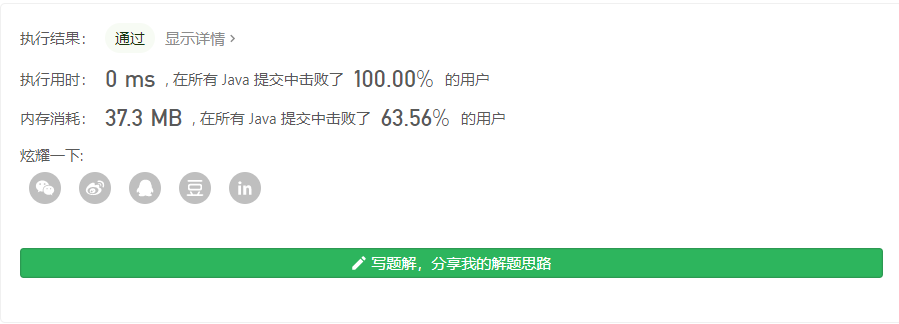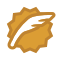# LeetCode 27移除元素&28实现strStr()&29两数相除

## 移除元素

// nums 是以“引用”方式传递的。也就是说，不对实参作任何拷贝
int len = removeElement(nums, val);
// 在函数里修改输入数组对于调用者是可见的。
// 根据你的函数返回的长度, 它会打印出数组中 该长度范围内 的所有元素。
for (int i = 0; i < len; i++) {
print(nums[i]);
}

ac代码为：

public int removeElement(int[] nums, int val) {
int index=0;

for(int i=0;i<nums.length;i++)
{
if(nums[i]==val)
continue;
nums[index++]=nums[i];
}
return index;
}## 实现 strStr()

public int strStr(String haystack, String needle) {
if(needle==null||"".equals(needle))
return 0;
char a[]=haystack.toCharArray();
char b[]=needle.toCharArray();
for(int i=0;i<a.length-b.length+1;i++)
{
int j=-1;
while(j++<b.length)
{
if(a[i+j]!=b[j])
{
break;
}
if(j==b.length-1)
return i;
}

}
return -1;
}public int strStr(String haystack, String needle) {
int L = needle.length(), n = haystack.length();
for (int start = 0; start < n - L + 1; ++start) {
if (haystack.substring(start, start + L).equals(needle)) {
return start;
}
}
return -1;
}


kmp方法笔者也实现了，但是由于数据问题效果一般般，当然kmp算法这里就不作详细介绍：

 public  int[] getNext(String needle) {
char[] p = needle.toCharArray();
int[] next = new int[p.length];
next = -1;
int j = 0;
int k = -1;
while (j < p.length - 1) {
if (k == -1 || p[j] == p[k]) {
next[++j] = ++k;
} else {
k = next[k];
}
}
return next;
}
public  int KMP(String haystack, String needle) {
char[] t = haystack.toCharArray();
char[] p = needle.toCharArray();
int i = 0; // 主串的位置
int j = 0; // 模式串的位置
int[] next = getNext(needle);
while (i < t.length && j < p.length) {
if (j == -1 || t[i] == p[j]) { // 当j为-1时，要移动的是i，当然j也要归0
i++;
j++;
} else {
// i不需要回溯了
// i = i - j + 1;
j = next[j]; // j回到指定位置
}
if(j==p.length) {return i-j;}
}
return -1;
}
public int strStr(String haystack, String needle) {
if(needle==null||"".equals(needle))
return 0;

return KMP(haystack, needle);
}public  int strStr(String haystack, String needle) {
if(needle==null||"".equals(needle))
return 0;
int lastchar[]=new int ;
char a[]=haystack.toCharArray();
char p[]=needle.toCharArray();
for(int i=0;i<p.length;i++)
{
lastchar[p[i]]=i+1;
}
int i=0;
int j=0;
int len=a.length-p.length+1;
while (i<a.length) {
int index=i-(lastchar[a[i]]-1);//a[i] 字符
if(lastchar[a[i]]!=0&&index>=0&&index<len)//可以匹配
{
while (j<p.length) {//尝试匹配
if(p[j]!=a[index+j])
break;
j++;
}
if(j==p.length)
{
return index;
}
else {
i=index+p.length;
j=0;
}
}
else {
i++;
}
}

return -1;
}## 两数相除

• 数值可能越界
• 不能使用乘除法public static int divide(int dividend, int divisor) {
int zhengfu=1;
long divd=dividend,divs=divisor;
if(dividend>0&&divisor<0)
{
divs=-divisor;zhengfu=-1;
}
else if(dividend<0&&divisor>0)
{
divd=-divd;zhengfu=-1;
}
else if (dividend<0&&divisor<0) {
divd=-divd;divs=-divs;
}

if(Math.abs(divd)<Math.abs(divs))return 0;
long i=0,index=0;
if(divs==1)i=divd+1;
else
while (index<=divd) {
i++;index+=divs;
}
long va=(zhengfu==1?(i-1):(1-i));
if(va>Integer.MAX_VALUE)return Integer.MAX_VALUE;
if(va<Integer.MIN_VALUE)return Integer.MIN_VALUE;
return (int) va;
}• 2=0010 表示有2个1
• 4=0100表示有4个1
• 6=0110表示有4+2个1public static int divide(int dividend, int divisor)
{
if(divisor==1)return dividend;
if(divisor==-1)return dividend==Integer.MIN_VALUE?Integer.MAX_VALUE:-dividend;

long value=0;//记录总次数结果
int time=1;//临时每次的次数
long divd=dividend,divs=divisor;//转成long处理

int zhengfu=1;//判断是正数还是负数
if(divd<0)
{
divd=-divd;zhengfu=-zhengfu;
}
if(divs<0)
{
divs=-divs;zhengfu=-zhengfu;
}
long team=divs;//临时数据2倍叠加
while (team<=divd) {
if(team+team>divd)
{
value+=time;
divd-=team;
team=divs;
time=1;

continue;
}
team+=team;
time+=time;

}
return (int) (zhengfu==1?value:-value);
}## 结语Big saiCSDN认证博客专家 scikit-learn

09-0158705-14173
12-09208
06-15253
11-15337
05-11305
03-292114
05-22570
05-04207
10-11248